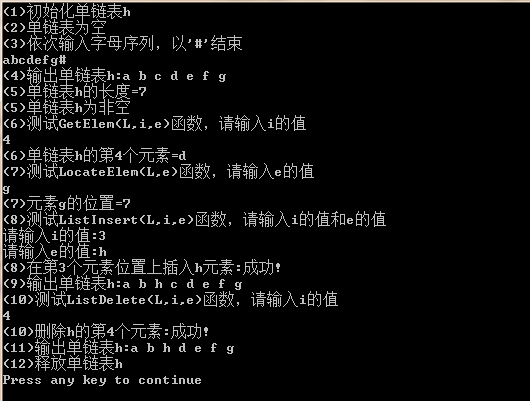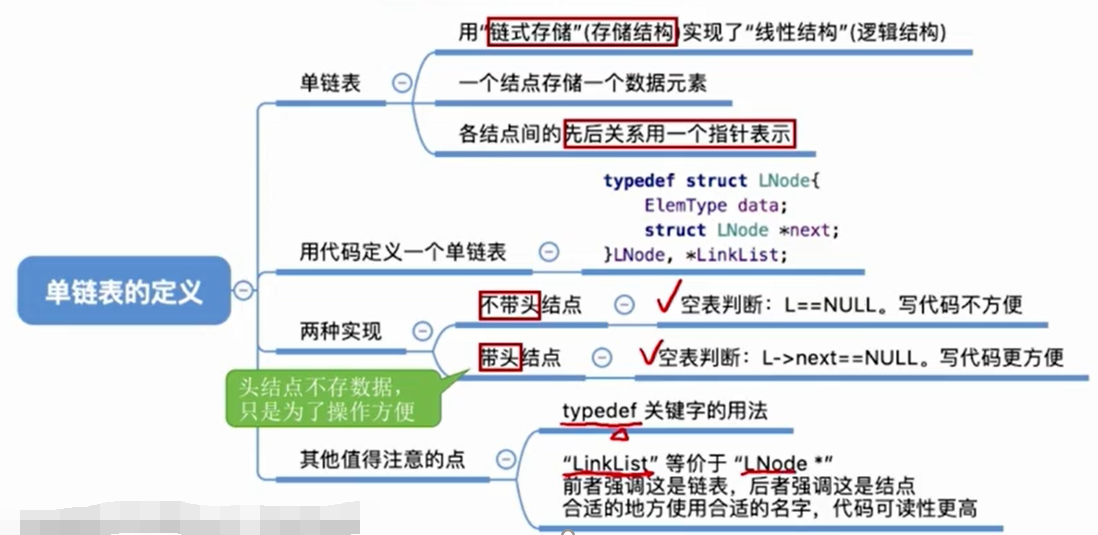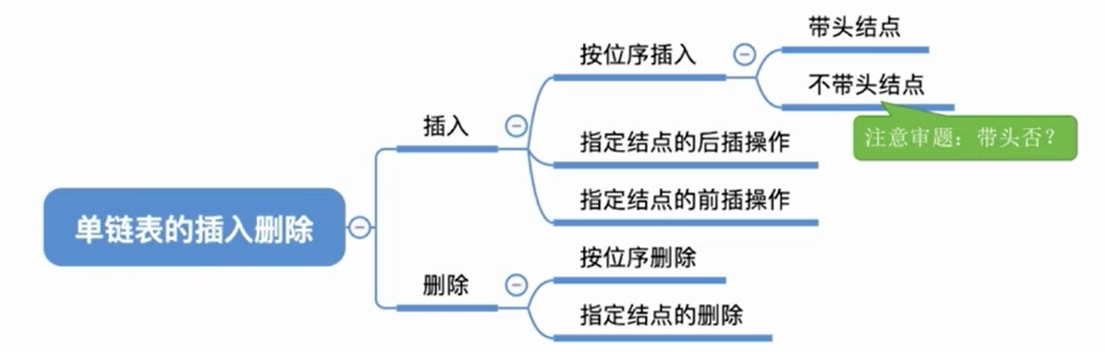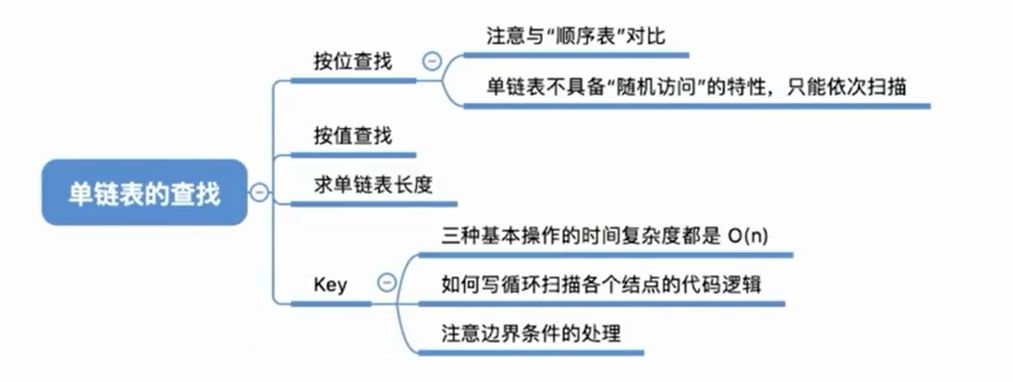• } 运行结果如下： C++线性表的链式存储结构 C++实现线性表链式存储结构: 为了解决顺序存储不足:用线性表另外一种结构-链式存储.在顺序存储结构(数组描述)中,元素的地址是由数学公式决定的,而在链式储存结构中,...

存档-----------

#include

typedef char ElemType;

void main()

{

ElemType e;

int i=;

int t=;

cout<

InitList(h);

cout<

cout<

cin>>e;

i=;

while(e!='#')

{

ListInsert(h,i,e);

i++;

cin>>e;

}

cout<

PrintList(h);

cout<

cout<

cout<

cin>>i;

t=GetElem(h,i,e);

if(t)

cout<

else

cout<

cout<

cin>>e;

t=LocateElem(h,e);

if(t)

cout<

else

cout<

cout<

cout<

cin>>i;

cout<

cin>>e;

cout<

t=ListInsert(h,i,e);

if(t)

cout<

else

cout<

cout<

PrintList(h);

cout<

cin>>i;

cout<

t=ListDelete(h,i,e);

if(t)

cout<

else

cout<

cout<

PrintList(h);

cout<

DestoryList(h);

}

typedef struct LNode//定义单链表结点类型

{

ElemType data;

struct LNode *next;

{

//初始化只含有头结点的空的单链表

L=new LNode;//创建头结点

if(L==NULL)

{

cout<

return ;

}

L->next=NULL;

return ;

}

{

//清空单链表，仅保留头结点

while(L->next)

{

p=L->next;

L->next=p->next;

delete p;

}

}

{

//返回单链表的长度

int i=;

while(p->next!=NULL)//数到最后一个结点为止

{

i++;

p=p->next;

}

return i;

}

{

//顺序输出单链表中的各元素

while(p!=NULL)

{

cout<data<

p=p->next;

}

cout<

}

{

//判断是否为空链表

if(L->next==NULL)

return true;

else

return false;

}

{

//用e返回单链表L中第i个元素的值

if(i

return ;

int j=;

while(j

{

p=p->next;

j++;

}

if(p==NULL)//j

return ;

else

{

e=p->data;

return ;

}

}

{

//返回e元素在单链表L中的位序，若不存在，返回0

int n=;

while(p!=NULL&&p->data!=e)

{

p=p->next;

n++;

}

if(p==NULL)//直到最后也没找到等于元素e的结点

return ;

else

return n;

}

{

//在单链表L的第i个数据元素之前插入数据元素e

if(i

return ;

int j=;//在1号位置插入时，i-1号位置是0号位置

while(j

{

p=p->next;

j++;

}

if(p==NULL)//未找到第i-1个结点，即i超出了[1..n+1]时

return ;

else//找到第i-1个结点p

{

s=new LNode;//创建新结点s

if(s==NULL)

{

cout<

return ;

}

s->data=e;

s->next=p->next;//将s插入到p之后

p->next=s;

return ;

}

}

{

//删除单链表L中第i个结点，并用e返回其值

if(i

return ;

int j=;

while(jnext)!=NULL)//寻找第i-1个结点，且第i-1号元素不是最后一个元素

{

p=p->next;

j++;

}

if((p->next)==NULL)//未找到第i-1个结点，即i超出了[1..n]

return ;

else//找到第i-1个结点p

{

q=p->next;//q指向要删除的结点

p->next=q->next;//从单链表中删除q结点

e=q->data;

delete q;//释放q结点

return ;

}

}

{

//销毁单链表

while(L)

{

p=L;

L=L->next;

delete p;

}

L=NULL;

}

运行结果如下：C&plus;&plus;线性表的链式存储结构

C++实现线性表的链式存储结构: 为了解决顺序存储不足:用线性表另外一种结构-链式存储.在顺序存储结构(数组描述)中,元素的地址是由数学公式决定的,而在链式储存结构中,元素的地址是随机分布的,每个元素 ...

C&plus;&plus;编程练习&lpar;2&rpar;----&OpenCurlyDoubleQuote;实现简单的线性表的链式存储结构&OpenCurlyDoubleQuote;

单链表采用链式存储结构,用一组任意的存储单元存放线性表的元素. 对于查找操作,单链表的时间复杂度为O(n). 对于插入和删除操作,单链表在确定位置后,插入和删除时间仅为O(1). 单链表不需要分配存储 ...

javascript实现数据结构：线性表--线性链表(链式存储结构)

上一节中, 线性表的顺序存储结构的特点是逻辑关系上相邻的两个元素在物理位置上也相邻,因此可以随机存取表中任一元素,它的存储位置可用一个简单,直观的公式来表示.然后,另一方面来看,这个特点也造成这种存储 ...

C 线性表的链式存储实现及插入、删除等操作示例

一.链式存储的优势 线性表的存储可以通过顺序存储或链式存储实现,其中顺序存储基于数组实现(见本人上一篇博客),在进行插入删除等操作时,需对表内某一部分元素逐个移动,效率较低.而链式结构不依赖于地址连续 ...

typedef struct LNode命名结构指针(线性表的链式存储)

一.typedef 关键字 1. 简介: typedef工具是一个高级数据特性,利用typedef可以为某一些类型自定义名称. 2. 工作原理: 例如我们定义链表的存储结构时,需要定义结点的存储数据元 ...

数据结构-线性表的链式存储相关算法(C语言实现)

链表的简单介绍 为什么需要线性链表 当然是为了克服顺序表的缺点,在顺序表中,做插入和删除操作时,需要大量的移动元素,导致效率下降. 线性链表的分类 按照链接方式: 按照实现角度: 线性链表的创建和简单 ...

线性表 顺序存储 链式存储 ---java实现

首先抽象出一个线性表抽象类(包括主要的增删操作) public abstract class MyAbstractList { public abstract void add(E ...

线性表的链式存储——C语言实现

SeqList.h #ifndef _WBM_LIST_H_ #define _WBM_LIST_H_ typedef void List; typedef void ListNode; //创建并且 ...

线性表的链式存储C语言版

#include #include #define N 10 typedef struct Node { int data; stru ...

随机推荐

Gridview样式的CSS控制

页面代码: .

toString结果

String[] str = new String[] { "a", "b", "c" }; System.out.println(str) ...

spring 配置文件 引入外部的property文件的两种方法

spring  的配置文件 引入外部的property文件的两种方法

展开全文• 1、链式存储：用一组任意的存储单元存储线性表, 逻辑上相邻的结点 在物理位置上不一定相邻，结点间的逻辑关系由存储结点时附加的指针字 段表示 2、链表：采用链式存储方法的线性表称为链表。 3、每一个结只包含一...

1、链式存储：用一组任意的存储单元存储线性表, 逻辑上相邻的结点 在物理位置上不一定相邻，结点间的逻辑关系由存储结点时附加的指针字 段表示

2、链表：采用链式存储方法的线性表称为链表。

3、每一个结只包含一个指针域的链表，称为单链表 为了正确表示结点间的逻辑关系，在存储每个结点值的同时，还必须 存储指示其直接后继结点的地址(或位置)，称为指针(pointer)或链(link)， 这两部分组成了链表中的结点结构 链表结点结构 data ：数据域，存放结点的值。next ：指针域，存放结点的直接后 继的地址。 带头结点的单链表的逻辑状态 物理存储方式 2.3.2 单链表的表示与操作 2.3.2.1 存储结构表示 结点是通过动态分配和释放来的实现，即需要时分配，不需要时释 放。实现时是分别使用C语言提供的标准函数：malloc() ，realloc()， sizeof() ，free() .

动态分配 p=(LNode*)malloc(sizeof(LNode)); typedef int ElemType; typedef struct Lnode { ElemType data; /*数据域，保存结点的值 */ struct Lnode *next; /*指针域*/ }LNode; /*结点的类型 */ 动态释放 free(p) ;

展开全文链表 数据结构
• 本文实例为大家分享了Java实现线性表链式存储，供大家参考，具体内容如下链表：一种物理存储结构上非连续、非顺序的存储结构,数据元素的逻辑顺序是通过链表中的指针链接次序实现的。package algorithm....

本文实例为大家分享了Java实现线性表的链式存储，供大家参考，具体内容如下

链表：一种物理存储结构上非连续、非顺序的存储结构,数据元素的逻辑顺序是通过链表中的指针链接次序实现的。

import java.util.NoSuchElementException;

/*

* 链表

* 物理存储上非连续的存储结构，数据元素的逻辑顺序是通过链表中的指针链接次序实现

*

* */

private int size;//链表长度

static private class Node{

private int data;

private Node next;

public Node(){

}

private Node(int data,Node next){

this.data=data;

this.next=next;

}

}

//初始化空链表

}

//添加元素

return true;

}

//在某个位置之前添加元素

checkPositionIndex(index);

if (index==size){

} else {

}

return true;

}

//根据下标获取元素

public int get(int index){

checkElementIndex(index);

return node(index).data;

}

//获取第一个元素

public Integer getFirst(){

if (f==null){

throw new NoSuchElementException();

} else {

return f.data;

}

}

//获取最后一个元素

public Integer getLast(){

if (size==0){

throw new NoSuchElementException();

}

int index=size-1;

return node(index).data;

}

//删除第一个元素

public Integer removeFirst(){

if (f==null){

throw new NoSuchElementException();

} else {

}

}

//删除最后一个元素

public Integer removeLast(){

if (size==0){

throw new NoSuchElementException();

}

int index=size-1;

}

//根据索引删除元素

public Integer remove(int index){

checkElementIndex(index);

}

//销毁链表

public void destroyList(){

clearList();

}

//将链表置为空表

public void clearList() {

Node next=p.next;//记录下一个结点

p=null;//删除当前结点

p=next;//指向下一个结点

}

size=0;

}

//遍历链表

public void traverseList(){

System.out.println(p.data);

p=p.next;

}

}

//返回链表元素个数

public int size(){

return size;

}

//尾部添加结点

Node cur =null,p;

if (size==0){//没有结点时

size++;

return;

}

cur=p;

p=cur.next;

}

cur.next= new Node(element,null);//尾部添加元素

size++;

}

//在某结点之前插入结点

if (node==null){

} else {

if (node.data==p.data){//node为结点时候

size++;

} else {

while (p!=null){

if (q.data==node.data) {

p.next= new Node(element,q);//在q之前(p之后)插入一个元素

size++;

break;

}

p=p.next;

q=p.next;

}

}

}

}

//删除结点

Integer deleteNodeData=null;

Node p=null;

deleteNodeData=node.data;

p=null;

size--;

} else {

for (p=q.next;p!=null;){//使用两个指针,p,q

if (p.data==node.data){

break;

}

q=q.next;//p始终为q的next结点

p=q.next;

}

q.next=p.next;

p=null;//删除p

size--;

}

return deleteNodeData;

}

//数组下标是否越界

private Boolean isElementIndex(int index){

return index>=0&&index

}

//插入位置是否越界

public Boolean isPositionIndex(int index){

return index>=0&&index<=size;

}

//检验下标是否越界，抛出异常

private void checkElementIndex(int index){

if(!isElementIndex(index)){

try {

throw new IndexOutOfBoundsException("下标越界");

} catch (Exception e) {

e.printStackTrace();

}

}

}

//检验插入下标是否越界，抛出异常

private void checkPositionIndex(int index){

if(!isPositionIndex(index)){

try {

throw new IndexOutOfBoundsException("下标越界");

} catch (Exception e) {

e.printStackTrace();

}

}

}

//返回指定位置的元素

private Node node(int index){

int nowIndex = 0;

if(size>0){

if (nowIndex==index){

return p;

}

p=p.next;

nowIndex++;

}

}

return null;

}

public static void main(String[] args) {

//测试

System.out.println("遍历链表");

System.out.println("遍历链表");

}

}

展开全文• 1.单链表基本知识点 2.单链表代码实现 1.带头结点（基本实现） ...1.头插法可以实现单链表 逆置 2. 偷天换日(见文末代码)-----在某个结点前插入结点 3.头插法和尾插法中心思想------- ... //存储数据 struct LNo.

0.概述
本文主要介绍单链表的基本知识，以及使用代码实现单链表的基本操作（增、删、改、查、头插法、尾插法等），阅读代码时应注意以下几点

1. 头插法可以实现单链表 逆置
2. 偷天换日(见下文第二段代码)-----在某个结点前插入结点
3. 头插法和尾插法中心思想------- 结点后插法 (见下文第一段代码)
4. 注意代码 封装 思想(见下文第二段代码)
5. 单链表 逆置代码实现 （见文末代码）

1.单链表基本知识点2.单链表代码实现

1.带头结点（基本操作代码实现）

#include<bits/stdc++.h>
using namespace std;

//定义结点
typedef struct LNode{
int data;	//存储数据
struct LNode *next;	//	存储下一个结点的指针

//0.初始化单链表
L=(LNode *)malloc(sizeof(LNode));
L->next=NULL;
}

//创建单链表核心思想——结点后插法
//1.尾插法
L->next=NULL;	//末尾指针指向null

LNode *s,*r=L;	//r始终指向最后一个结点 //声明的是指针变量

//核心思想——结点后插法 （在r后插入结点s）
for(int i=0;i<n;i++){
s=(LNode *)malloc(sizeof(LNode));	//为指针变量分配存储空间
s->data=a[i];
s->next=r->next;
r->next=s;
r=s;
}
return L;
}

//2.头插法——可以实现单链表逆置
L->next=NULL;

LNode *s;	//声明的是指针变量
//核心思想——结点后插法
for(int i=0;i<n;i++){
s=(LNode *)malloc(sizeof(LNode));	//为指针变量分配存储空间
s->data=a[i];
s->next=L->next;
L->next=s;
}
return L;
}

//1.插入
if(i<1){
return false;
}
LNode *p;	//指针p指到当前扫描到的节点
int j=0;	//指针p当前扫描到的位置
p=L;	//p指向头节点，头节点是第0个节点

while(p!=NULL&&j<i-1){	//	找到第i-1个位置
p=p->next;
j++;
}

if(p==NULL){	//	检查i的值，即找到表末尾也未找到i-1的位置
return 0;
}

LNode *s=(LNode *)malloc(sizeof(LNode));
s->data=e;

//以下两个顺序不能颠倒
s->next=p->next;
p->next=s;

return true;
}

//2.删除
bool Delete(LinkList &L,int i,int &e){		//&e带回删除的值
if(i<1){
return false;
}
LNode *p;
int j=0;
p=L;
while(p!=NULL&&j<i-1){
p=p->next;
j++;
}

if(p==NULL){	//判断i的值是否合法
return false;
}

LNode *s=(LNode *)malloc(sizeof(LNode));	//方便释放结点空间
s=p->next;
e=s->data;	//存储要删除结点的值
p->next=s->next;
free(s);
return true;
}

//3.按位查找
if(i<1){
return NULL;
}
LNode *p=L;		//指向正在扫描的结点
int j=0;	//正在扫描的结点为第几个结点
while(p!=NULL&&j<i){
p=p->next;
j++;
}
return p;
}

//4.按值查找
LNode *p=L;	//	指向第一个存有数据的节点
int j=0;

while(p->next!=NULL){
p=p->next;
j++;
if(p->data==e){
return j;	//查找成功，返回位置
}
}

return 0;
}

//5.单链表长度
LNode *p=L;
int cnt=0;
while(p->next!=NULL){
p=p->next;
cnt++;
}
return cnt;
}

//6.循环遍历输出
LNode *p=L;
while(p->next!=NULL){
p=p->next;
cout<<p->data<<" ";
}
cout<<endl;
}

//主程序
int main(){

int a[]={1,2,3,4,5,6};
//0.测试头插法 和尾插法 遍历输出
//	PrintList(L);

L=TailCreate(a,6);
PrintList(L);

//1.测试插入
//	if(Insert(L,3,8)){
//		PrintList(L);
//	} else{
//		cout<<"插入异常！！！"<<endl;
//	}

//2.测试删除
//	int e=-1;
//	if(Delete(L,3,e)){
//		PrintList(L);
//		cout<<e<<endl;
//	} else{
//		cout<<"删除异常！！！"<<endl;
//	}

//3.测试按位查找
//	LNode *p=Get(L,3);
//	cout<<p->data<<endl;

//4.测试按值查找
//	int i=Locate(L,3);
//	cout<<i<<endl;

//5.测试单链表长度
//	int len=Length(L);
//	cout<<len<<endl;

return 0;
}

2.结点后插法 偷天换日 封装思想

//指定结点的后插操作
bool InsertNextNode(LNode *p,int e){
if(p==NULL){
return false;
}
LNode *s=(LNode *)malloc(sizeof(LNode));
s->data=e;
s->next=p->next;
p->next=s;
return true;
}

//指定结点的前插入操作——偷天换日
bool InsertPriorNode(LNode *p,int e){
if(p==NULL){
return false;
}
LNode *s=(LNode *)malloc(sizeof(LNode));

//换值，并交换p、s结点的位置
s->data=p->data;
s->next=p->next;
p->next=s;
p->data=e;
return true;
}

// 删除指定结点——偷天换日
bool DeleteNode(LNode *p){
if(p==NULL){
return false;
}

//含有小bug,不能删除指定的表末最后一个结点
LNode *s=p->next;
p->data=s->data;
p->next=s->next;
free(s);
return true;
}

//封装思想测试
int main(){

int a[]={1,2,3,4,5,6};
//0.尾插法 遍历输出
L=TailCreate(a,6);
PrintList(L);

//6.在封装的基础上，测试插入
//6.1查找i-1的位置
LNode *p=Get(L,2);	//按位查找结点
//6.2在第i-1个节点后插入元素
if(InsertNextNode(p,8)){	//偷天换日
PrintList(L);
} else{
cout<<"插入异常！！！"<<endl;
}

return 0;
}

3.单链表逆置代码实现

//7.逆置单链表
L1->next=NULL;

LNode *s;	//作为新链表的新结点

LNode *p=L->next;	//	扫描原链表的结点

for(int i=1;i<=n;i++){	//i可以表示扫描到原来链表的位置
s=(LNode *)malloc(sizeof(LNode));
s->data=p->data;
s->next=L1->next;
L1->next=s;

//释放原来链表结点
s=p;
p=p->next;
free(s);	//释放扫描后的结点
}
return L1;
}

//主程序
int main(){

int a[]={1,2,3,4,5,6};
//0.头插法创建单链表 遍历输出
L=TailCreate(a,6);
PrintList(L);

//7.单链表逆置---逆置单链表L
int len=Length(L);
L=InversionList(L,Length(L));
PrintList(L);

return 0;
}

展开全文数据结构 单链表 c++
• 2线性表的链式存储及其实现虽然顺序表具有随机存取的特点是一种有用的存储结构但是也有缺陷...表一旦形成就很少进行插入操作，对于要进行平凡的插入或者删除操作的“动态的”线性表通常采用链式存储结构，与此同时链...
• 用Python 来实现 C语言中 线性表链式存储结构。代码地址# !/usr/bin/env python# -*- coding: utf-8 -*-__author__ = 'MrHero'class SqList(object):def __init__(self, size):self.data = list(None for _ in ...
• 线性表链式存储结构线性表实现分顺序存储结构和链式存储结构。上一节我们学学习了线性表实现分顺序存储结构，并实现解顺序存储的基本操作。这一节我们来学习线性表链式存储结构，那我们再想象一下我为什么我们...
• 线性表）试编写算法将线性表就地逆置，以链式存储结构实现。 代码： #include <stdio.h> #include <malloc.h> struct Num { int n; struct Num *next; }num; struct Num *createlist(struct Num *...链表 算法 数据结构
• 文章目录1.单链表的建立1.1头插法1.2尾插法2.链表基本操作 1.单链表的建立 ...//存储后继节点位置的指针域，用next表示 }LinkNode; 头插法代码如下，由数组元素a创建单链表L void CreatListF(Lin数据结构
• * 链表储存结构实现顺序表 * @param <E> */ public class LinkedList<E> { // 头节点 private LinkedNode<E> first; // 尾节点 private LinkedNode<E> last; // 顺序表内储存的数据...java 链表 数据结构数据结构 链表
• 1、记录自己学习数据结构中对于链式表的实现实现了对于链式表的增插删改查 2、其中最难的是插入和删除因为该操作都涉及到了指针的指向问题， 插入函数ListInsert_L代码片段+详解 Status ListInsert_L(LinkList&...数据结构 链表 c语言
• ​ 在学数据结构的时候，第一次上机实验，老师让我们实现线性表的顺序存储结构和链式存储结构及其简单操作，看着就脑阔疼。书上的代码吧，都是伪代码，照着敲出来n多报错（我们用的是清华大学出版社严蔚敏、陈文博...
• class SequenceList():def __init__(self):self.SeqList = []def createSequenceList(self):element = input('please input a numer and enter # ensure:')while element != '#':self.SeqList.append(int(element))e...
• c++ 实现线性表 数组 #include <iostream> #include "stdlib.h" using namespace std; #define MAXSIZE 20 //设置存储空间初始分量 #define OK 1 #define ERROR 0 typedef int ElemType;//ElemType 相当与int ...c++ 数据结构 链表
• 链式存储是最常用的动态存储方法，通常将采用链式存储结构线性表称为线性链表。从链接方式看，链表可分为单链表、循环链表和双链表。从实现角度看，链表可分为动态链表和静态链表。 链表用一组任意的存储单元来...数据结构 c语言 链表c语言 数据结构 线性表的链式存储结构
• 1. 线性表（Linear List）: 由同类型数据元素构成有序序列的线性结构 表中元素个数称为线性表的长度 线性表没有元素时，成为空表 表起始位置称表头，结束位置称表尾 ...※ 线性表链式存储 实现： 不要求逻辑数据结构 链表
• 这里给出线性表的顺序存储和链式存储 链式存储的主要优点是便于数据修改，相对于顺序存储来说，主要缺点是存储空间的利用效率较低， 因为分配给元素的存储单位有一部分用来存储相邻结点的存储地址，由于链式存储...
• C语言手写线性表 顺序存储结构、链式存储结构C语言手写线性表(顺序存储结构、链式存储结构)顺序存储结构（通过数组实现链式存储结构（结构体实现） C语言手写线性表(顺序存储结构、链式存储结构) 顺序存储结构...指针 链表 数据结构 单链表 算法
• 文章目录前言一、线性表链式存储结构实现1.实现所需要的主函数2.常量定义3.定义结构体4.实现4.1单链表的初始化4.2单链表的建立-头插法4.3单链表的建立-尾插法（带头结点）4.4单链表的清空4.5获取单链表的长度4.6...c语言 数据结构 链表
• 链式存储结构：解决顺序存储结构的不足之处 结点：数据域(存储数据元素本身信息的域)+指针域(存储直接后继元素位置的域) 头指针： 链表必要元素，指向第一个结点的指针，若链表有头结点，则是指向头结点的指针。 ...
• 文章目录前言一、顺序存储结构1：建立一个包含数组的结构体2：获得元素（从数组中获取所需元素）3：插入元素4：删除元素二、链式存储结构（方法1）1.创建承载数据的载体2:创建链表2.显示链表数据3：删除链表数据4：...数据结构 链表 指针 c++ c语言
• 线性表的顺序存储结构以图书管理系统为例（C语言版） 一、线性表的顺序存储结构 这里用一张图进行说明。灵魂画手作画，并不是在实际内存中的准确形式，只是用来帮助理解。 二、具体代码实现 1.调用头文件以及宏定义 ...数据结构 c语言...